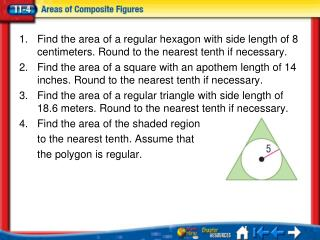Download PresentationLesson 4 Menu - PowerPoint PPT Presentation

Download PresentationAn Image/Link below is provided (as is) to download presentation

Download Policy: Content on the Website is provided to you AS IS for your information and personal use and may not be sold / licensed / shared on other websites without getting consent from its author. While downloading, if for some reason you are not able to download a presentation, the publisher may have deleted the file from their server.

- - - - - - - - - - - - - - - - - - - - - - - - - - - E N D - - - - - - - - - - - - - - - - - - - - - - - - - - -
Presentation Transcript

1. Find the area of a regular hexagon with side length of 8 centimeters. Round to the nearest tenth if necessary. • Find the area of a square with an apothem length of 14 inches. Round to the nearest tenth if necessary. • Find the area of a regular triangle with side length of 18.6 meters. Round to the nearest tenth if necessary. • Find the area of the shaded region to the nearest tenth. Assume that the polygon is regular. Lesson 4 Menu

2. Find areas of composite figures. • Find areas of composite figures on the coordinate plane. • composite figure Lesson 4 MI/Vocab

3. Lesson 4 PS1

4. Area of a Composite Figure What is the area of the composite figure? Round to the nearest tenth. A 713.1 ft2 B 852.5 ft2 C 953.1 ft2 D 992 ft2 Read the Item The figure can be separated into a rectangle with dimensions 16 feet by 32 feet, a triangle with a base of 32 feet and a height of 15 feet, and two semicircles with radii of 8 feet. Lesson 4 Ex1

5. Area of a Composite Figure Solve the Item Area of composite figure Area formulas Substitution Simplify. Use a calculator. Answer: The area of the composite figure is 953.1 square feet to the nearest tenth. The correct answer is C. Lesson 4 Ex1

6. Find the area of the figure in square feet. Round to the nearest tenth if necessary. • A • B • C • D A. 478.5 ft2 B. 311.2 ft2 C. 351.2 ft2 D. 438.5 ft2 Lesson 4 CYP1

7. Find the Area of a Composite Figure to Solve a Problem A rectangular rose garden is centered in a border of lawn. Find the area of the lawn around the garden in square feet. The length of the entire lawn is 25 + 100 + 25 or 150 feet. The width of the entire lawn is 25 + 20 + 25 or 70 feet. The length of the rose garden is 100 feet and the width is 20 feet. Lesson 4 Ex2

8. area of composite figure – area of rose garden Find the Area of a Composite Figure to Solve a Problem Area formulas Substitution Simplify. Simplify. Answer: The area of the lawn around the garden is 8500 square feet. Lesson 4 Ex2

9. INTERIOR DESIGN Cara wants to wallpaper one wall of her family room. She has a fireplace in the center of the wall. Find the area of the wall around the fireplace. • A • B • C • D A. 168 ft2 B. 156 ft2 C. 204 ft2 D. 180 ft2 Lesson 4 CYP2

10. First, separate the figure into regions. Draw an auxiliary line perpendicular to from M (we will call this point S), an auxiliary line from N to the x-axis (we will call this point K), and an auxiliary line from P to the Origin, O. Coordinate Plane Find the area of polygon MNPQR. This divides the figure into triangle MRS, triangle NKM, trapezoid POKN and trapezoid PQSO. Lesson 4 Ex3

11. Coordinate Plane Now, find the area of each of the figures. Find the difference between y-coordinates to find the lengths of the bases of the triangles and the lengths of the bases of the trapezoids. Find the difference between x-coordinates to find the heights of the triangles and trapezoids. Lesson 4 Ex3

12. Δ Δ Coordinate Plane Area formulas Substitution Simplify. Answer: The area of polygon MNPQR is 44.5 square units. Lesson 4 Ex3

13. Find the area of polygon ABCDE. • A • B • C • D A. 38 units2 B. 17 units2 C. 15 units2 D. 19 units2 Lesson 4 CYP3